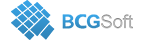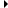BCGControlBar Pro for MFC
CBCGPChartMACDFormula

Detailed Description

The formula is displayed as a histogram series and represents difference between slow and fast EMA. To show signal line you need to create an MA formula for MACD series.

The following example illustrates how to create MACD formula applied to close values of stock series with signal line:

Copy
// MACD
CBCGPChartMACDFormula macd(12, 26);
CBCGPChartSeries* pMACDSeries = macd.Create(pChart, _T("MACD"), pCloseSeries, pAxisMACD);
pMACDSeries->m_bIncludeSeriesToLegend = FALSE;
// MACD Signal
CBCGPChartMAFormula signalMACD(CBCGPChartMAFormula::MA_SIMPLE, 9);
CBCGPChartSeries* pSignalMACDSeries = signalMACD.Create(pChart, _T("Signal MACD"), pMACDSeries, pAxisMACD);
pSignalMACDSeries->m_bIncludeSeriesToLegend = FALSE;
// where pAxisMACD is Y axis of MACD series.Inheritance diagram for CBCGPChartMACDFormula:

enum  ValueType

Public Member Functions

CBCGPChartMACDFormulaConstructs a CBCGPChartMACDFormula object.

CalculateDataPointCalculates a formula data point at the specified index in the input series.

GetFastEMAPeriodReturns fast EMA period.

GetSlowEMAPeriodReturns slow EMA period.

GetValueTypeReturns the formula calculation type.

SetPeriodsSets slow and fast EMA periods.

SetValueTypeSets a formula calculation type.Public Member Functions inherited from CBCGPChartAdvancedFormula
CreateCreates a formula.

GeneratePointsCalled to generate data points for an output series.

GetOutputSeriesCategoryReturns output series category.

GetOutputSeriesTypeReturns output series type.

GetPeriodReturns formula period.

SetOutputSeriesCategorySets output series category.

SetOutputSeriesTypeSets output series type.

SetPeriodSets formula period.Public Member Functions inherited from CBCGPChartBaseFormula
FindInputSeriesIndexFinds input series index.

GetInputSeriesAtReturns an input series located at the specified position.

GetInputSeriesCountReturns the number of input series.

GetLParamReturns user-defined data.

GetParentSeriesReturns a pointer to a parent series.

IsNonDiscreteCurveTells whether the formula is displayed as a non-discrete curve.

SetLParamSets user-defined data.

SetParentSeriesSets a parent series that represents a formula on the diagram.Public Attributes inherited from CBCGPChartAdvancedFormula